View Perfect Parallelepipeds 1 and 5

by Jorge Sawyer and Cliff Reiter

The 1st Example with max Edge length 271

In:=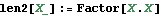In:=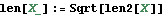In:=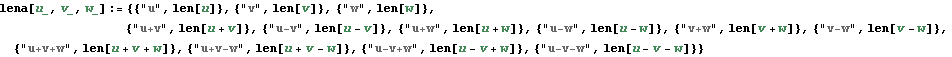In:=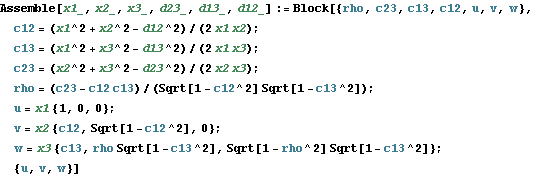In:=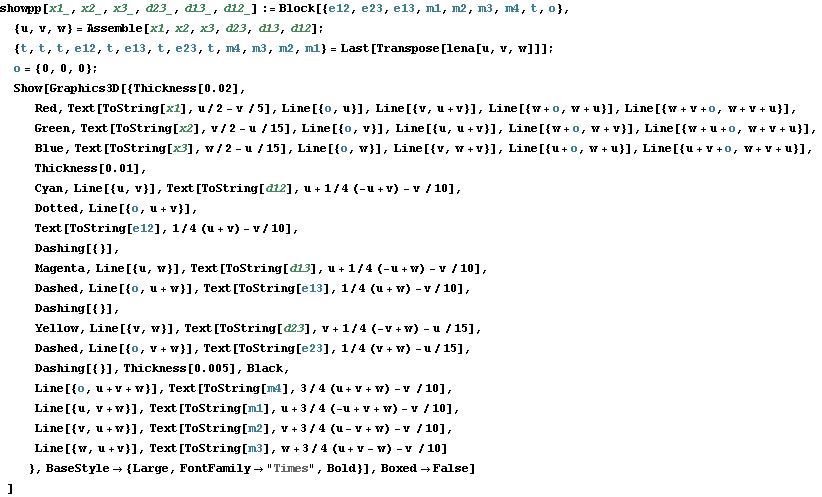Example 1

In:=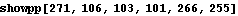Out=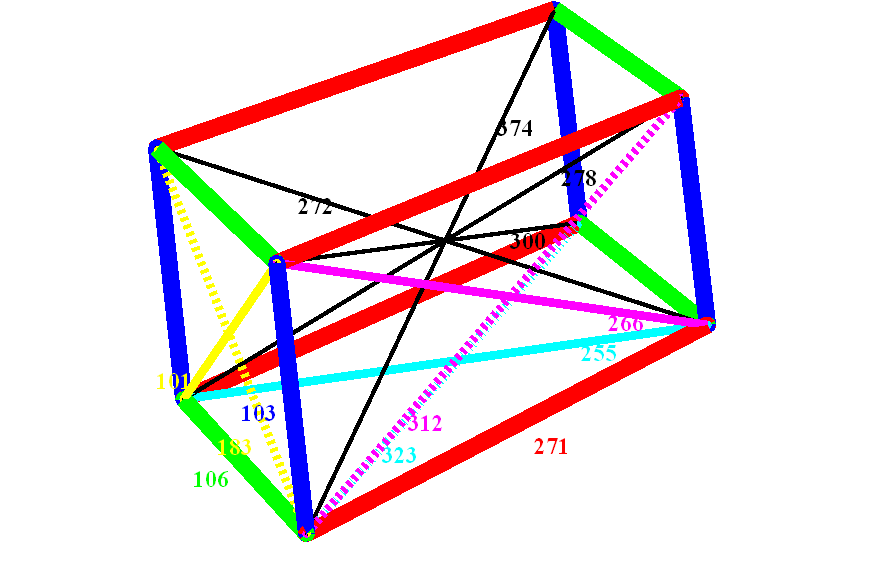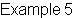In:=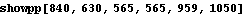Out=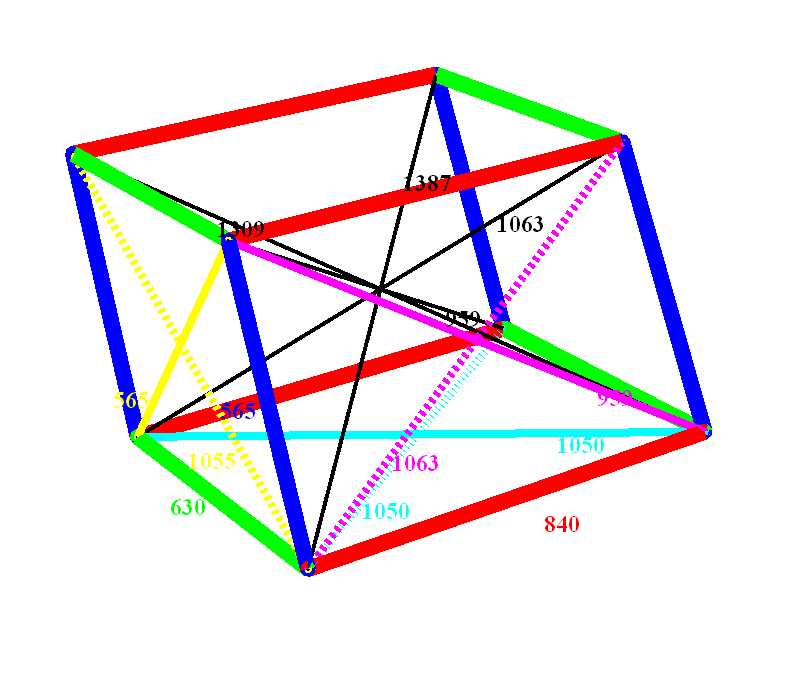Created by Wolfram Mathematica 6.0  (24 July 2009)